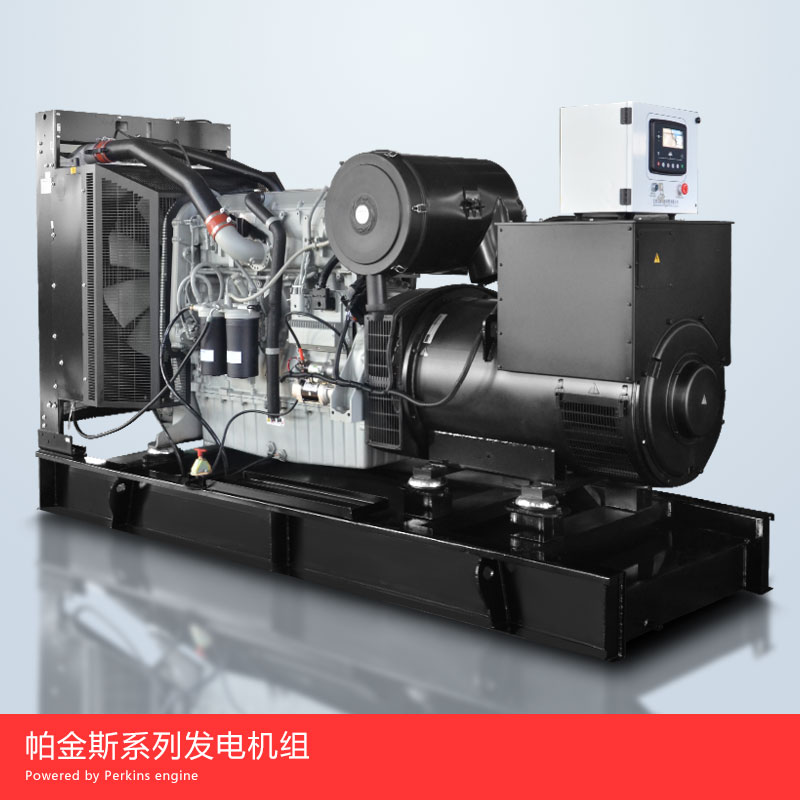139-5261-9321

# 发电机组油耗参考值

30kW油耗量=6.3公斤（kg）=7.8升（L）

45kW油耗量=9.45公斤（kg）=11.84升（L）

50kW油耗量=10.5公斤（kg）=13.1升（L）

75kW油耗量=15.7公斤（kg）=19.7升（L）

100kW油耗量=21公斤（kg）=26.25升（L）

150kW油耗量=31.5公斤（kg）=39.4升（L）

200kW油耗量=40公斤（kg）=50升（L）

250kW油耗量=52.5公斤（kg）=65.6升（L）

300kW油耗量=63公斤（kg）=78.75升（L）

350kW油耗量=73.5公斤（kg）=91.8升（L）

400kW油耗量=84.0公斤（kg）=105升（L）

450kW油耗量=94.5公斤（kg）=118升（L）

500kW油耗量=105公斤（kg）=131.2升（L）